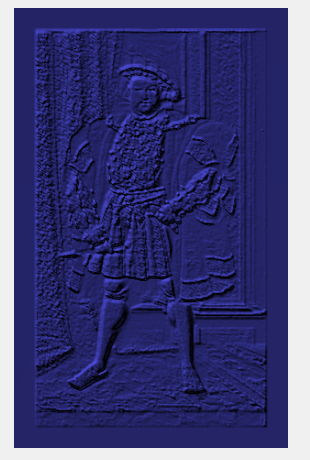OK, 3D here we come

You’ll note that in the last post, we converted the image to monochrome, but the final step was left tantalisingly empty:

function setLevels(heightData, width, height) {
// TODO - create 3D data from height data
}

So, let’s finish off and populate the function to produce a lithophane.

I’m going to use the 3D JavaScript library three.js, it’s easy to use and open source, so it’s a good candidate and will allow us to display and manipulate the 3D structures required.

There is a type of geometry in three.js called ParametricGeometry that lends itself very well  to our task.  ParametricGeometry is an object that creates a planar structure like a terrain with a set width and depth, but calls a function for each pixel to determine the height of each each point on that plane like a 3D landscape with hills and valleys.

lithoFace=new THREE.ParametricGeometry(getPoint, width, height);

The function called (for each point in the plane), takes two arguments, u and v, each of these is a value between 0 and 1 where 0 is the far left/top of the image and 1 is the far right/bottom. Given these parameters, the function should create and return a 3D vector containing the x, y and z co-ordinates of the referenced point.

function getPoint(u,v) {
var x=width*u;
var y=height*v;
// use the height data collected from the image
// to return a height for each pixel
return new THREE.Vector3 (x,y,heightData[width*y+x]);
}

lithoFace now contains the geometry of our negative monochrome image and can be displayed by three.js (if a material is added):

var lithoMaterial = new THREE.MeshBasicMaterial( { color: 0x3030C0 });
var lithoMesh = new THREE.Mesh (lithoFace ,lithoMaterial);

Which gives us a beautiful 3 Dimensional lithophane of Holbein’s masterpiece:flipping this over (as we want to print it in negative and have the light shining through it) and adding 5 more planes to this face. Making it an enclosed box and then use the internal structures of the Geometry to export a simple ASCII .STL string that can be saved to a file:

function vertexAsString(vert){
return vert.x+" "+vert.y+" "+vert.z;
}
function generateSTL(geometry,name) {
var vertices = geometry.vertices;
var faces = geometry.faces;
var stl = "solid "+name+"\n";
for(var i = 0; i<faces.length; i++){
stl += ("facet normal "+vertexAsString( faces[i].normal )+" \n");
stl += ("outer loop \n");
stl += "vertex "+vertexAsString( vertices[ faces[i].a ])+" \n";
stl += "vertex "+vertexAsString( vertices[ faces[i].b ])+" \n";
stl += "vertex "+vertexAsString( vertices[ faces[i].c ])+" \n";
stl += ("endloop \n");
stl += ("endfacet \n");
}
stl += ("endsolid "+name+"\n");
return stl;
}

This string can be passed back to the browser as if a download link has been clicked to save the .stl to the local hard disk

function saveSTL( geometry, name ){
var stlString = generateSTL( geometry,name );

var blob = new Blob([stlString], {type: 'text/plain'});

saveAs(blob, name + '.stl'); // add the .STL extension
}
function saveAs(blob,name) {
if (window.webkitURL !== null) {
// Chrome allows the link to be clicked
// without actually adding it to the DOM.
}
else {
// before it can be clicked.
}
}

That’s it! You have a simple program written in JavaScript that runs in your browser and operates on local files.

The finished version allows you to drag an image from the desktop and sends back the Lithophane as an STL to your download folder.

https://3dp.rocks/lithophane/

Please give it a try and let me know what you think.

I’ll be tackling placing the image on the surface of a shape other than a plane in another post, so come back for more soon…MakeALot

1.William says: